# Trapezoid

The trapezoid is fascinating because it is defined based on the geography you belong to.

If you’re visiting the United Kingdom on an exchange trip and ask a student to draw a trapezoid for you, then s/he will draw it like a trapezium.

The trapezoid has its opposite sides parallel to each other, which is how you calculate its area.

A trapezoid (trapezium) is a quadrilateral in which one pair of opposite sides are parallel.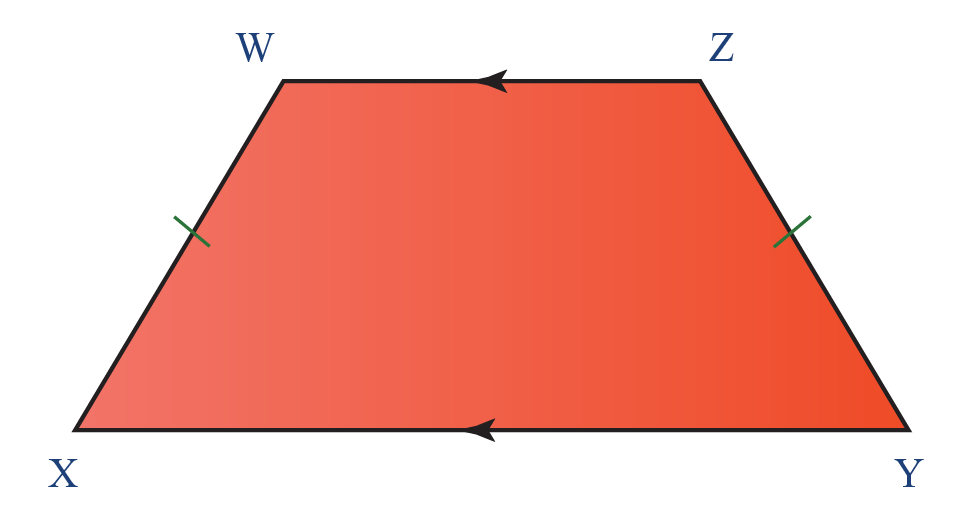In the above diagram $$XY$$ and $$WZ$$ are parallel to each other and are called bases

Meanwhile, the sides that are not parallel to each other are called legs. In the above image, $$WX$$ and $$YZ$$ are the legs of the trapezoid.

Some real life examples of trapezoids include the following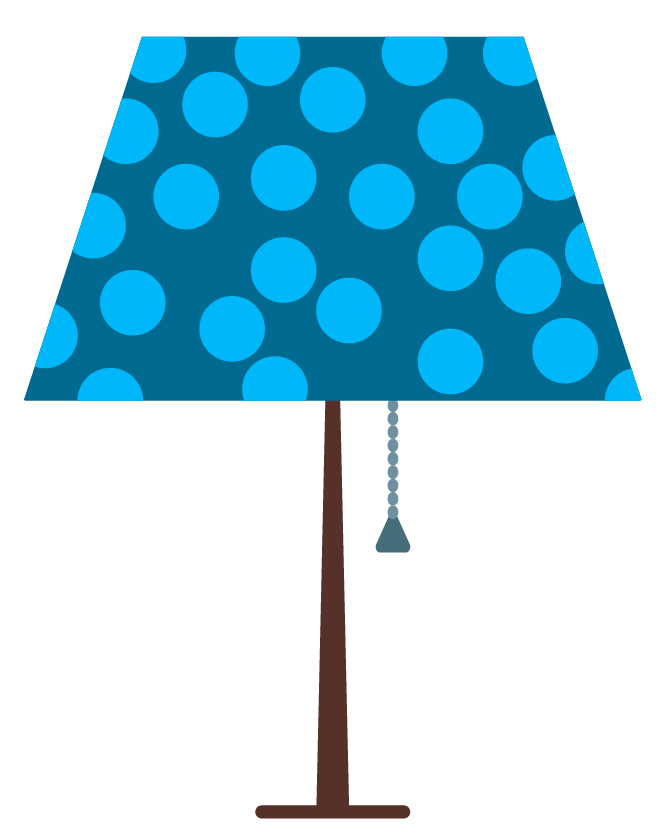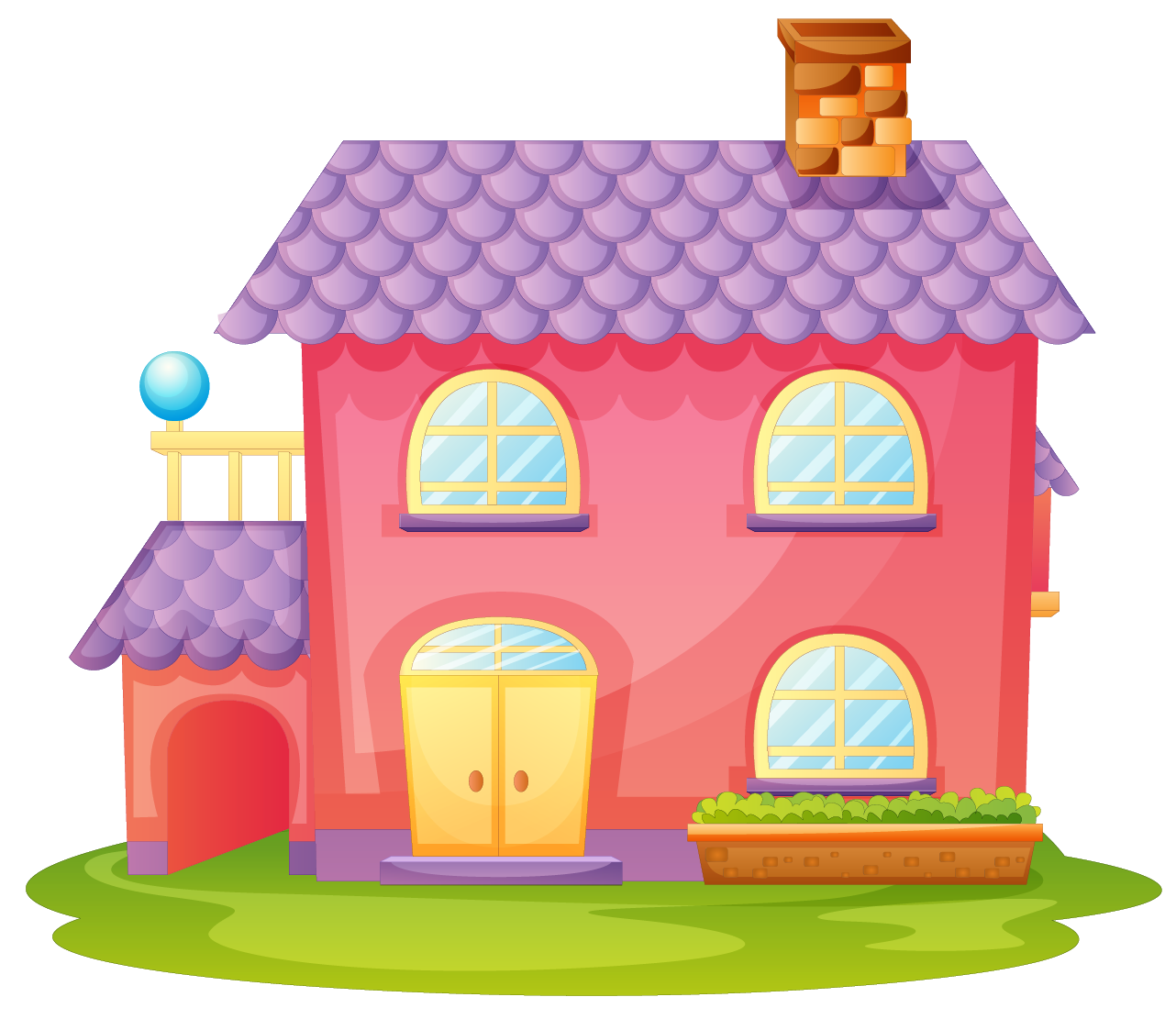## Lesson Plan

 1 What is a Trapezoid? 2 Think Tank 3 Solved Examples on Trapezoid 4 Interactive Questions on Trapezoid 4 Tips and Tricks

## What is a Trapezoid's Properties?

These are the properties of a trapezoid that make it stand out from other quadrilaterals

1. The bases (the top and bottom) are parallel to each other
2. Opposite sides of a trapezoid (isosceles) are the same length
3. The angles on either side of the base are congruent
4. Diagonals are equal in length
5. Angles next to each other sum up to $$180^\circ$$

## Types of trapezoids

There are three types of trapezoids

1. Isosceles Trapezoid
2. Scalene Trapezoid
3. Right Trapezoid

If the legs of the trapezoid are equal in length, then it is called an isosceles trapezoid.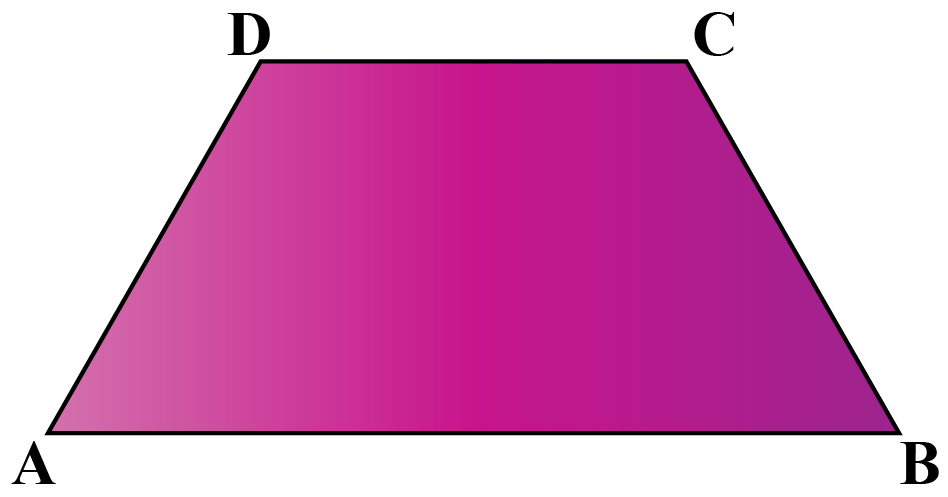When neither the sides nor the angles of the trapezoid are equal, then it is a scalene trapezoid.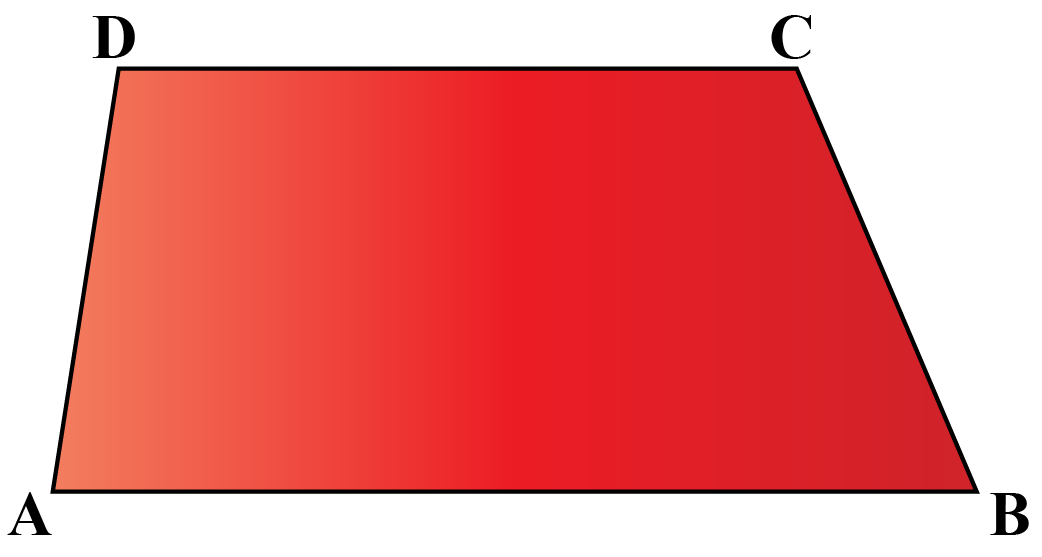A right trapezoid has a pair of right angles.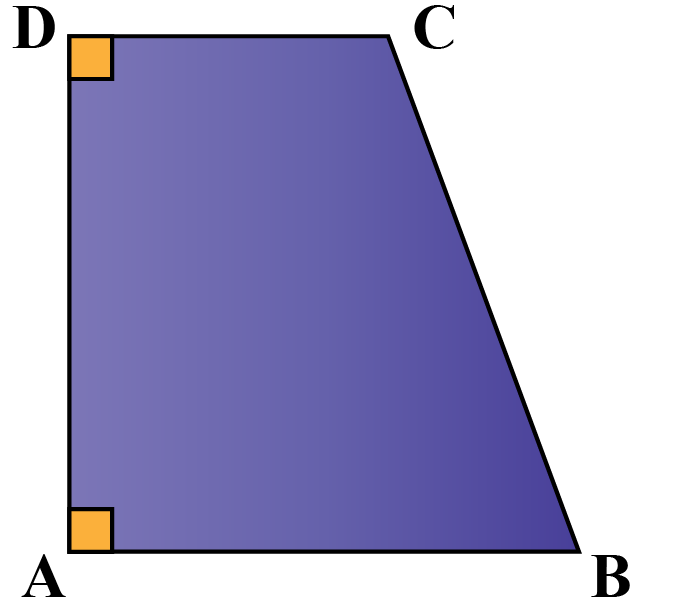## Area and Perimeter of a Trapezoid

The area of the trapezoid is calculated by measuring the average of the parallel sides and multiplying it with its height.

The area of a trapezoid is calculated by the following formula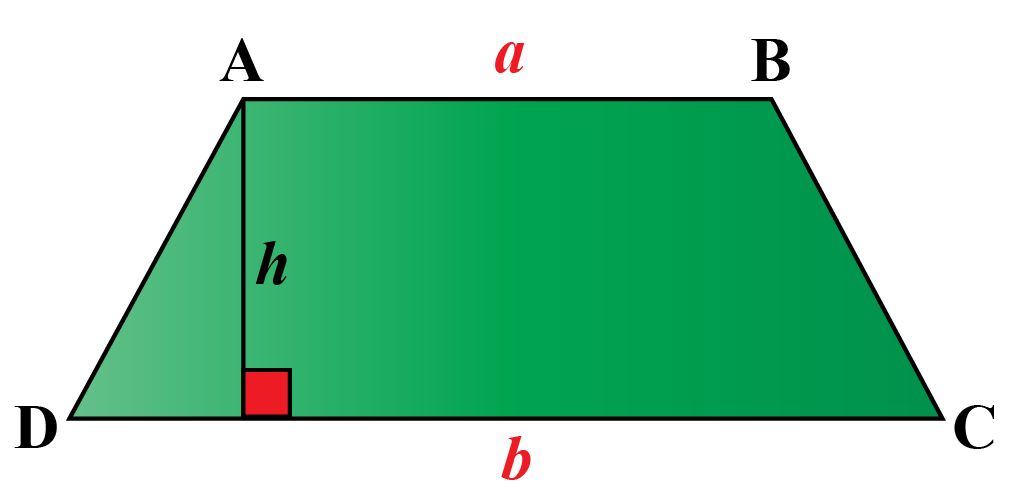$A = \dfrac{{AB+CD }}{2}\times DE$

$$\text {Where AB} \text { and CD}$$ are the parallel sides and $$DE$$ is the height

The perimeter of a trapezoid is the sum of all its sides.

Therefore a trapezoid with sides $$AB, BC, CD \text{ and}\text { AD}$$ has a perimeter formula that can be written as

$Perimeter = AB + BC + CD + AD$Think Tank
1. Find the area of the shaded region in green where $$PQ= 40 \text { cm}, RS=30 \text { cm}$$, and the shortest distance between $$PQ \text { and} \text { RS} \text { is} \text { 28 cm}$$.
Taking $$P,Q,R \text { and}\text { S}$$ as the centers, four arcs of circles of radius $$14 cm$$ each are drawn in the following figure.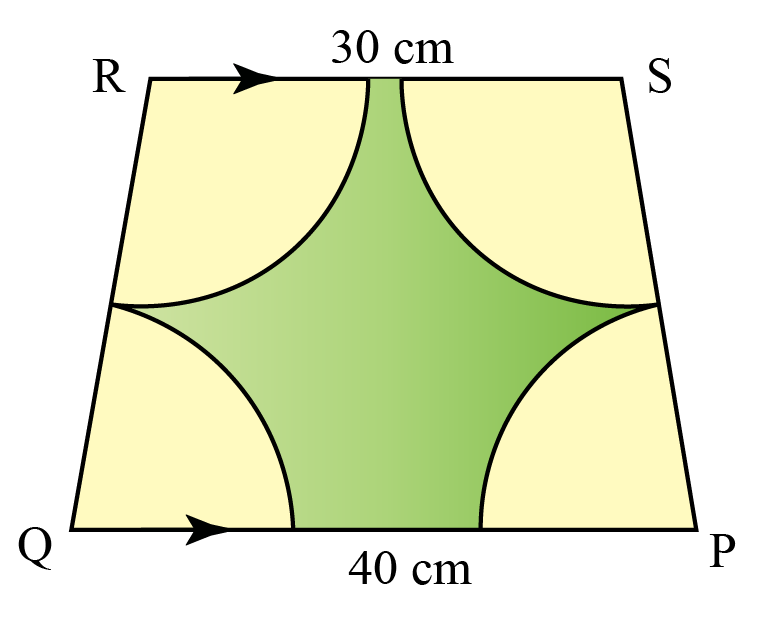## Solved Examples

 Example 1

Can you help Donald find the height of the trapezium if he is given the area and the lengths of the two parallel sides. The area is $$128 inches^2$$. If the lengths of the bases are $$12 inches$$ and $$20 inches$$.

Solution

Let us assume that the bases are $$a$$ and $$b$$; and the height of the trapezoid is  $$h$$

Using the given information,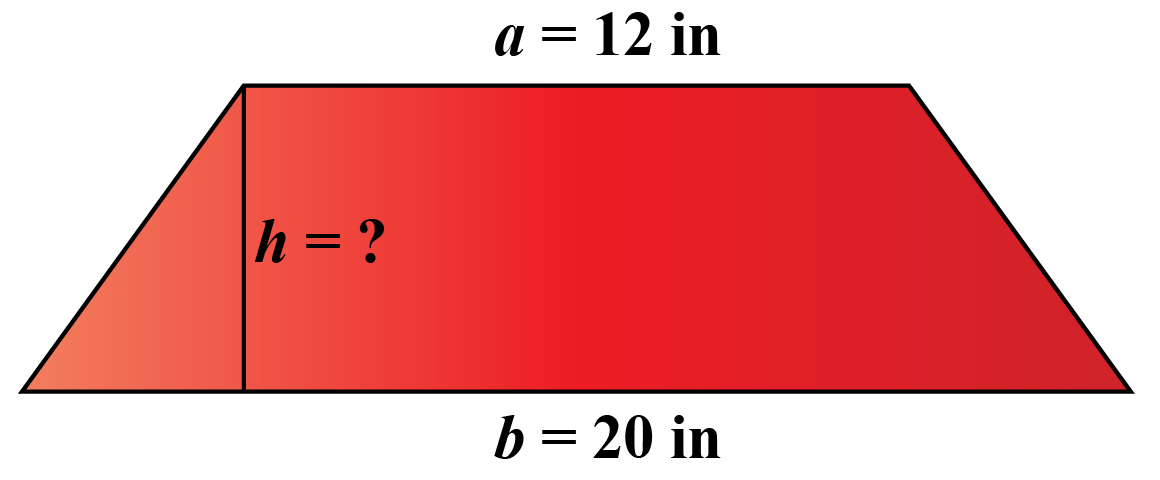We have to find $$h$$ which is the distance or height between the bases.

Let us substitute all these values in the area of a trapezoid formula:

\begin {align} A &= \dfrac{{a+b }}{2}\times h\\ 128 &= \dfrac{{20+12}}{2}\times h\\ 256 &= 32\times h\\ h &= 8 \text{ inches}\end{align}

 $$\therefore$$ Distance between the bases is $$8\text{ inches}$$
 Example 2

Sarah has been asked to find the area of a trapezoid that has the following values. Can you help her?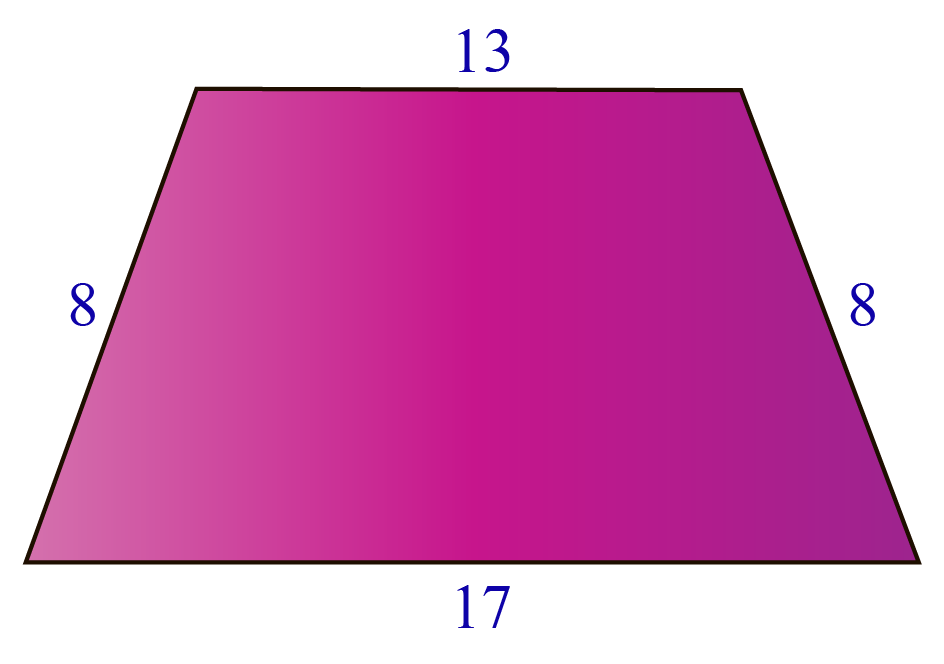Solution

Let us assume that $$a$$ and $$b$$are the bases, and $$h$$ is the height of the given trapezoid.

The above trapezoid can be represented as follows: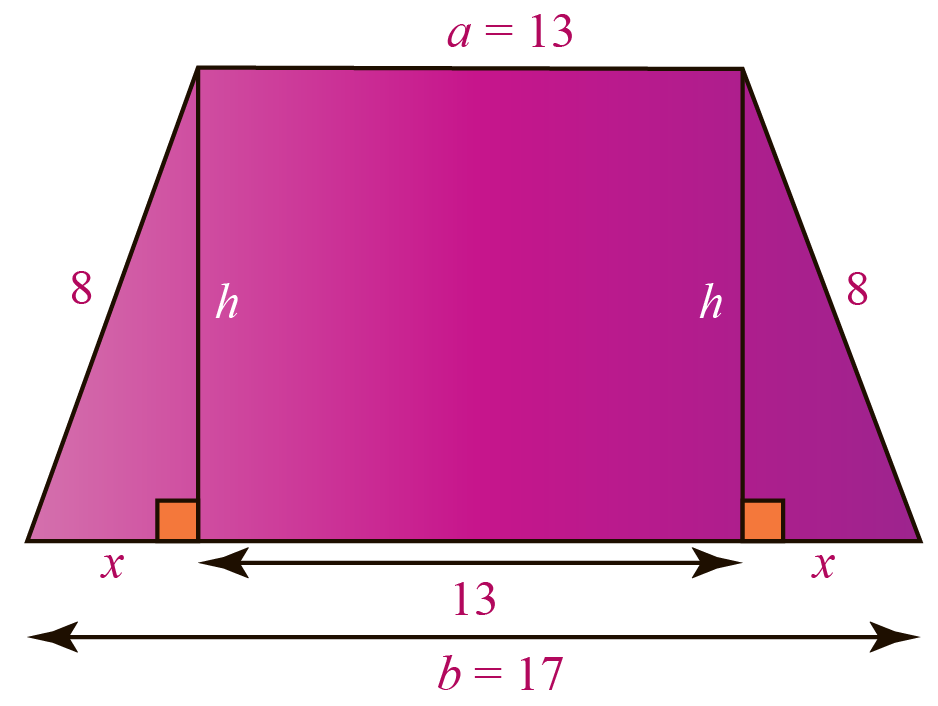\begin {align} 13 + x + x &= 17\\ 13 + 2x &=17\\ 2x &= 4\\ \therefore x &=2 \end{align}

Now, applying Pythagoras' Theorem to one of the triangles,

\begin {align} 8^2 &= 2^2 + h^2\\ 64&= 4 + h^2\\ h^2 &= 60\\ h &= \sqrt{60}\\ h &= \sqrt {4\times 15}\\ h &= 2 \sqrt {15} \end{align}

Finally, we will use the area of a trapezoid formula to find its area:

\begin {align} A &= \dfrac{{a+b }}{2}\times h\\ A &= \dfrac{{13+17}}{2}\times 2 \sqrt {15}\\ A &= 60 \sqrt {15} \div 2\\ A &= 30 \sqrt {15} \text{ sq units}\end{align}

 $$\therefore \text { A} = 30\sqrt {15} \text { sq units}$$
 Example 3

Find the area of the front face $$ABCD$$ of the following prism.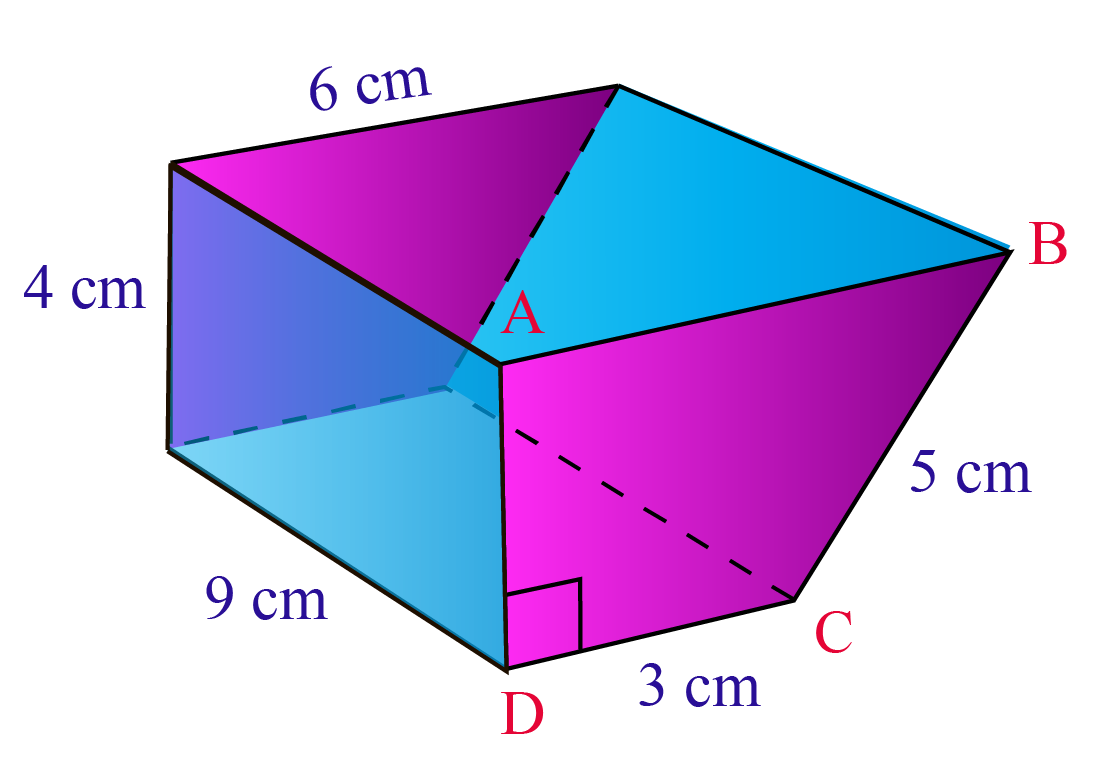Solution

From the given figure, we can find the length of the bases and the height of the trapezoid.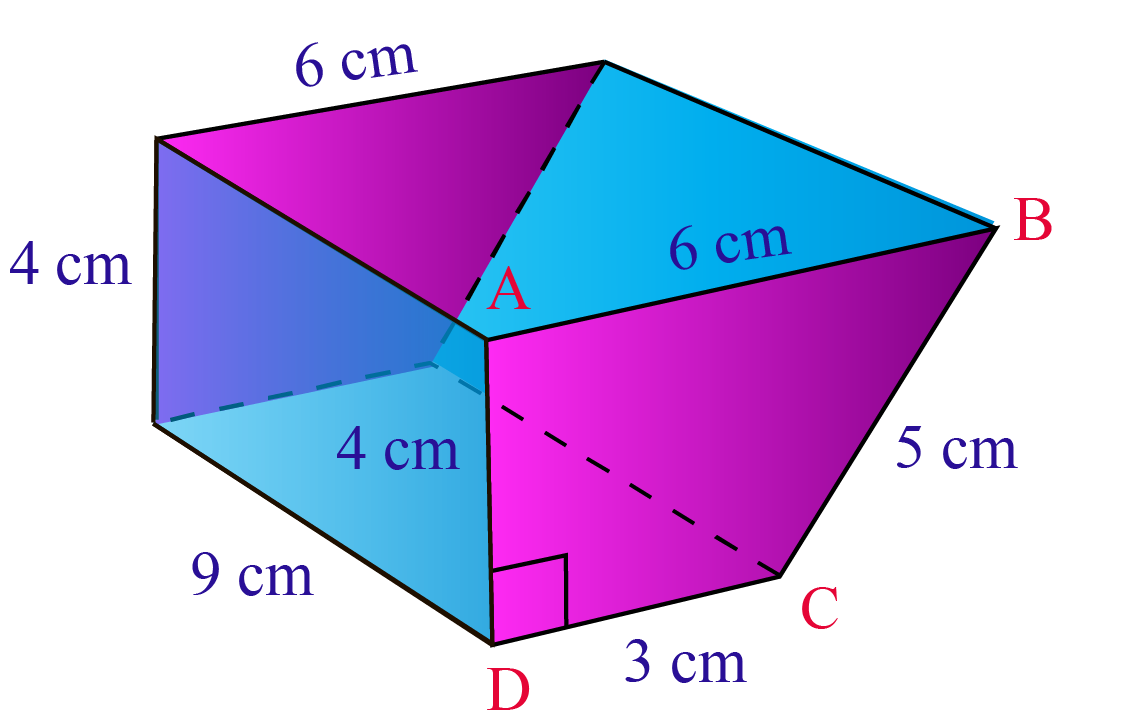The parallel sides $$AB$$ and $$CD$$ are $$6\text{ cm}$$ and $$3\text{ cm}$$ respectively

From the details given in the diagram, we can deducce that the height $$AD = 4\text { cm}$$

The area of the trapezoid is calculated using the formula:

\begin {align} A &= \dfrac{{AB+CD }}{2}\times AD\\ A&= \dfrac{6+3}{2}\times 4\\ A &= 18 \text { cm} \end {align}

 $$\therefore A = 18 \text { sq cm}$$
 Example 4

Jason has been told that the length of one of the parallel sides of a trapezoid is $$6 inches$$. Its height is $$4\text inches$$, and its area is $$32\text { inches^2}$$. Help him find the length of the other side.

Solution

From the data given to Jason, here's what the trapezoid looks like: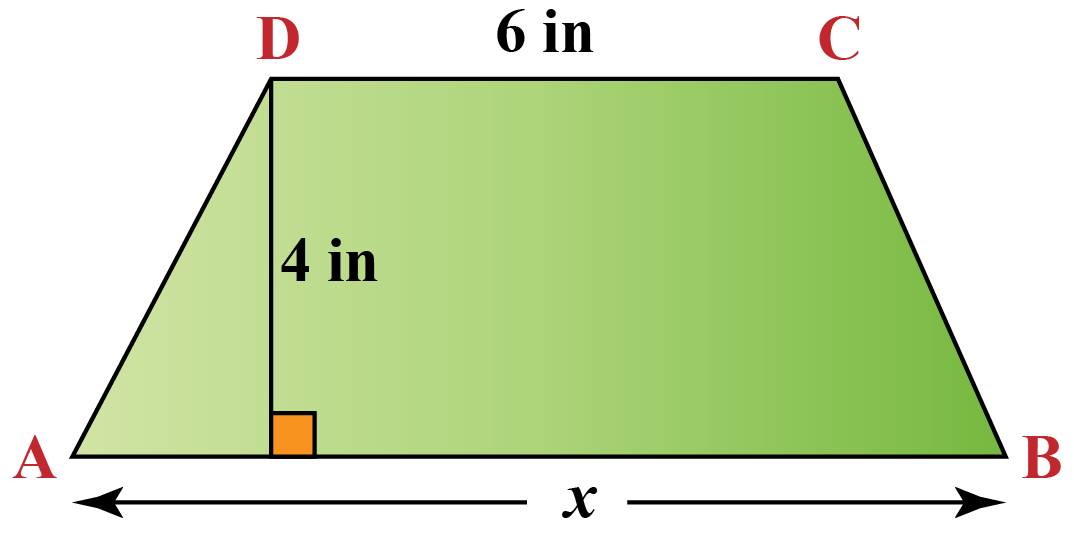\begin {align} A &= \dfrac{{AB+CD }}{2}\times AD\\ 32&= \dfrac{AB+6}{2}\times 4\\ 64 &= AB + 6 \times 4\\ 16 &= AB+6\\ AB&=10 \text { cm} \end {align}

 $$\therefore AB = 10 \text { inches}$$
 Example 5

Can you help Charlotte find the area of a trapezoid with bases of 3 meters and 5 meters and a height of 4 meters.

Solution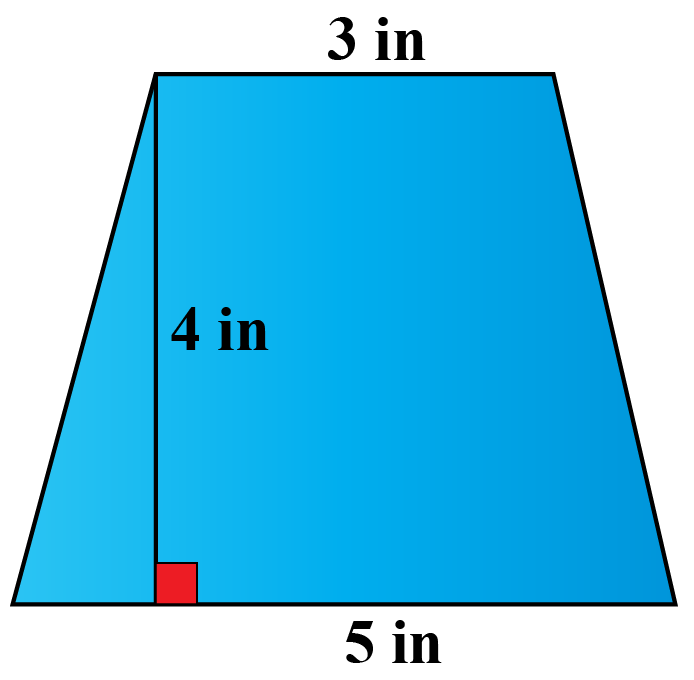\begin {align} A &= \dfrac{{AB+CD }}{2}\times AD\\ A&= \dfrac{3+5}{2}\times 4\\ A &= 8 \times 2\\ A &= 16 \ m^2 \end {align}

 $$\therefore A = 16 m^2$$

## Interactive Questions

Here are a few activities for you to practice. Select/Type your answer and click the "Check Answer" button to see the result.Tips and Tricks
1. A trapezoid an isosceles trapezoid when it has equal angles from a parallel side
2. It is called a trapezium in the United Kingdom and other parts of the world

## Let's Summarize

The mini-lesson targeted the fascinating concept of trapezoids. The math journey around trapezoids starts with what a student already knows, and goes on to creatively crafting a fresh concept in the young minds. Done in a way that not only it is relatable and easy to grasp, but also will stay with them forever. Here lies the magic with Cuemath.

At Cuemath, our team of math experts is dedicated to making learning fun for our favorite readers, the students!

Through an interactive and engaging learning-teaching-learning approach, the teachers explore all angles of a topic.

Be it worksheets, online classes, doubt sessions, or any other form of relation, it’s the logical thinking and smart learning approach that we, at Cuemath, believe in.

## 1. How to find the area of a trapezoid?

The area of a trapezoid is calculated by calculating the average of the two parallel sides and multiplying it with its height

$A = \dfrac{{PQ+RS }}{2}\times ST$

## 2. What is the equation for a trapezoid?

There are two equations for a trapezoid. One equation calculates its area; the other its perimeter. The area of a trapezoid is as explained in the equation above.

The perimeter of a trapezoid $$PQRS$$ is given as
$$Perimeter$$ = $$PQ$$ + $$QR$$ + $$RS$$ + $$PS$$

## 3. Is trapezoid a quadrilateral?

Since the trapezoid has four sides, it automatically becomes a quadrilateral. It has two sides that are parallel, an two sides that aren’t.

## 4. What are 3 attributes of a trapezoid?

The 3 main attributes of a trapezoid are as follows

1. Its base angles and diagonals are equal if the trapezoid is isosceles
2. The intersection point of the diagonals is collinear (in the same line) to the midpoints of the two opposite sides
3. Opposite sides of an isosceles trapezoid are congruent

## 5. How do you find the missing side of a trapezoid?

The missing side of the trapezoid can be determined from the information given to you. In case, you have the area, and the length of one side, you can find the length of the missing side. You can also determine the length of the missing side if you know the perimeter, and the length of three sides of the trapezoid.

More Important Topics
Numbers
Algebra
Geometry
Measurement
Money
Data
Trigonometry
Calculus